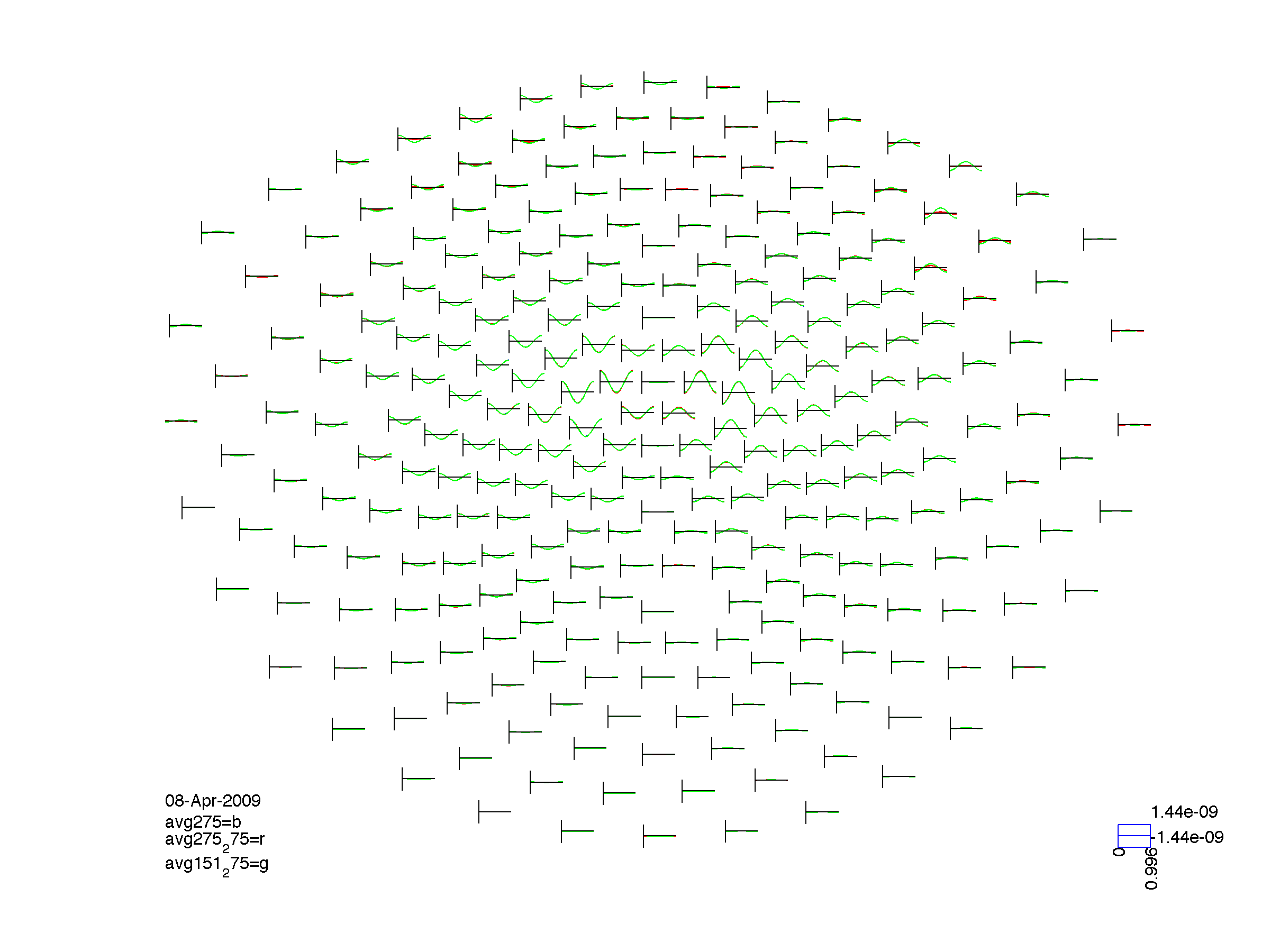Tags: example meg ctf

# Interpolating data from the CTF151 to the CTF275 sensor array using megrealign

``````% the two example files with the 151 and 275 channel CTF specifications are available from
% ftp://ftp.fieldtriptoolbox.org/pub/fieldtrip/example/megrealign/

vol = [];
vol.r = 12;
vol.o = [0 0 4];

% in this example the dipole is carefully positioned on the same depth as
% where the dipole layer for the interpolation will be located. The center
% of the head is at [0 0 4], the radius is 12 cm, and the dipole layer that is
% specified in ft_megrealign is 2.5 cm shifted inward from the head surface
cfg = [];
cfg.dip.pos = [0 0 13.5];  % 4 + 12 - 2.5
cfg.dip.frequency = 1;
data151 = ft_dipolesimulation(cfg);
data275 = ft_dipolesimulation(cfg);

cfg = [];
avg151 = ft_timelockanalysis(cfg, data151);
avg275 = ft_timelockanalysis(cfg, data275);

% here I'll not only realign the 151 channel MEG data to the 275 channel and
% vice versa, but also apply the ft_megrealign function from 151 to 151. In the
% realignment the signal will be slightly distorted as a comparison of avg151_151
% to avg151 would show.
cfg = [];
cfg.inwardshift = 3;
cfg.template{1} = grad151; avg151_151 = ft_timelockanalysis([], ft_megrealign(cfg, avg151));
cfg.template{1} = grad275; avg151_275 = ft_timelockanalysis([], ft_megrealign(cfg, avg151));
cfg.template{1} = grad151; avg275_151 = ft_timelockanalysis([], ft_megrealign(cfg, avg275));
cfg.template{1} = grad275; avg275_275 = ft_timelockanalysis([], ft_megrealign(cfg, avg275));

% plot the realigned datasets
cfg = [];
figure; ft_multiplotER(cfg, avg151_151);
title('Interpolated from 151 to 151');
orient portrait; print -dpng -r300 avg151_151.png
``````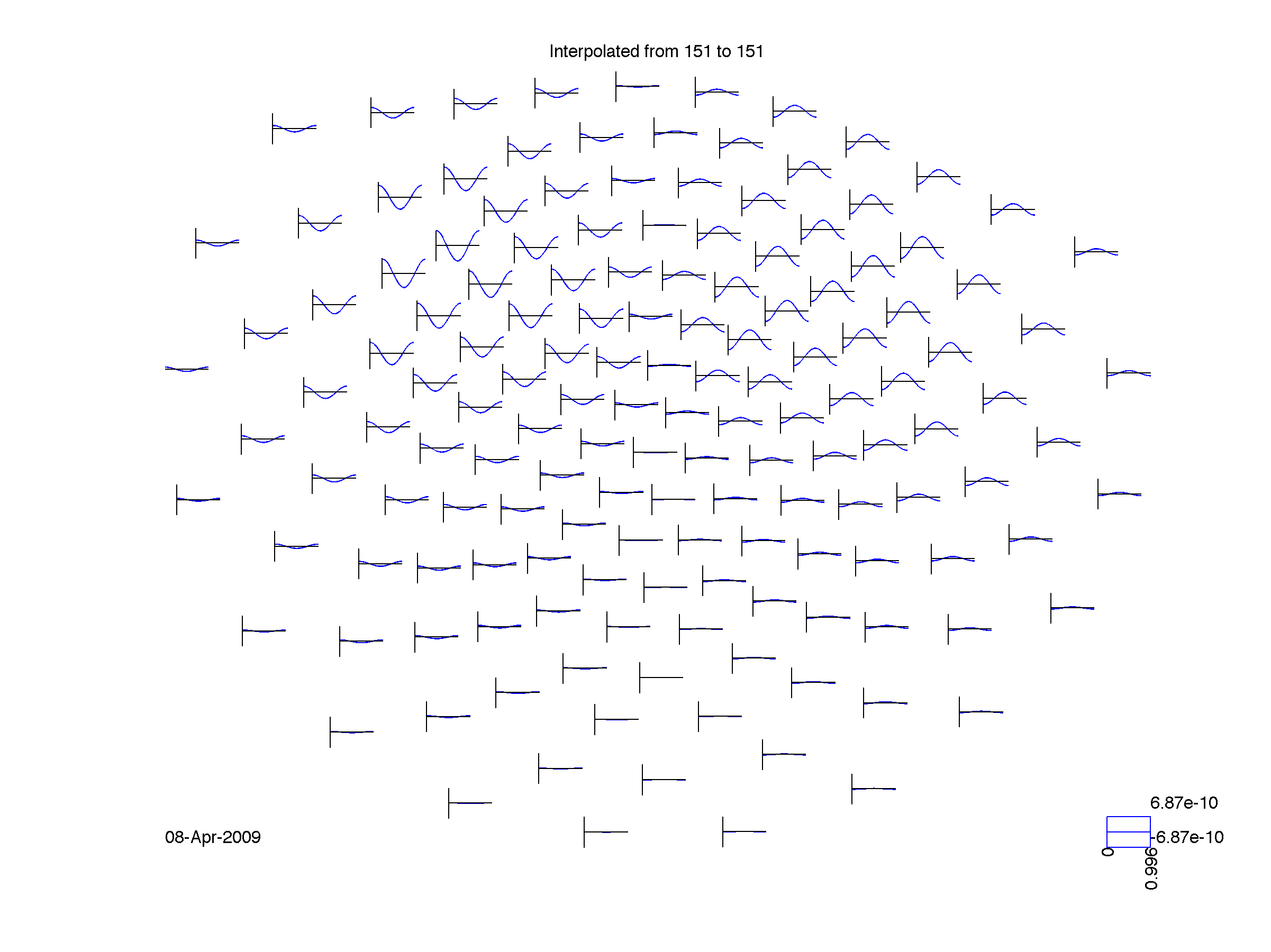``````figure; ft_multiplotER(cfg, avg151_275);
title('Interpolated from 151 to 151');
orient portrait; print -dpng -r300 avg151_275.png
``````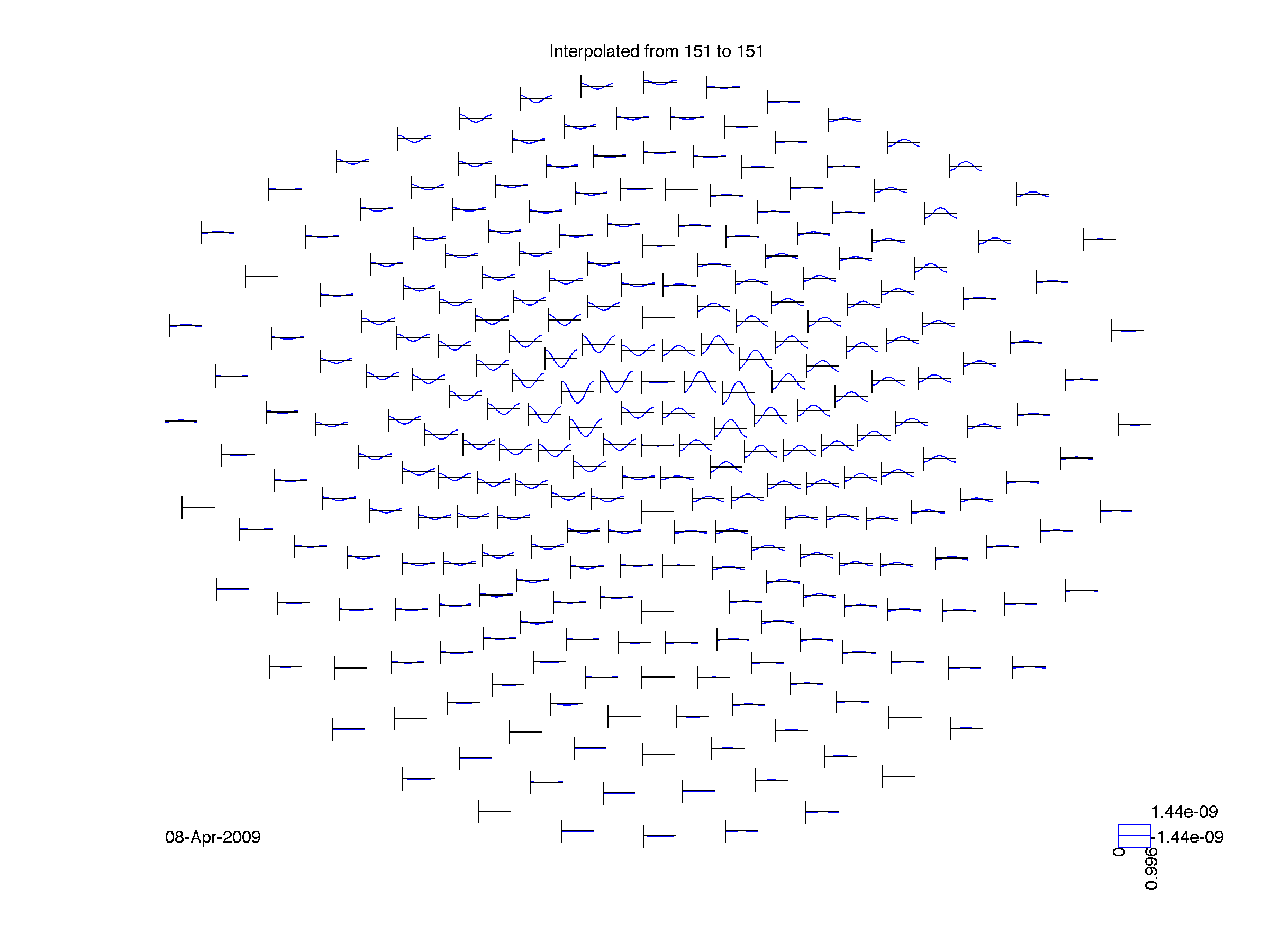``````figure; ft_multiplotER(cfg, avg275_151);
title('Interpolated from 151 to 151');
orient portrait; print -dpng -r300 avg275_151.png
``````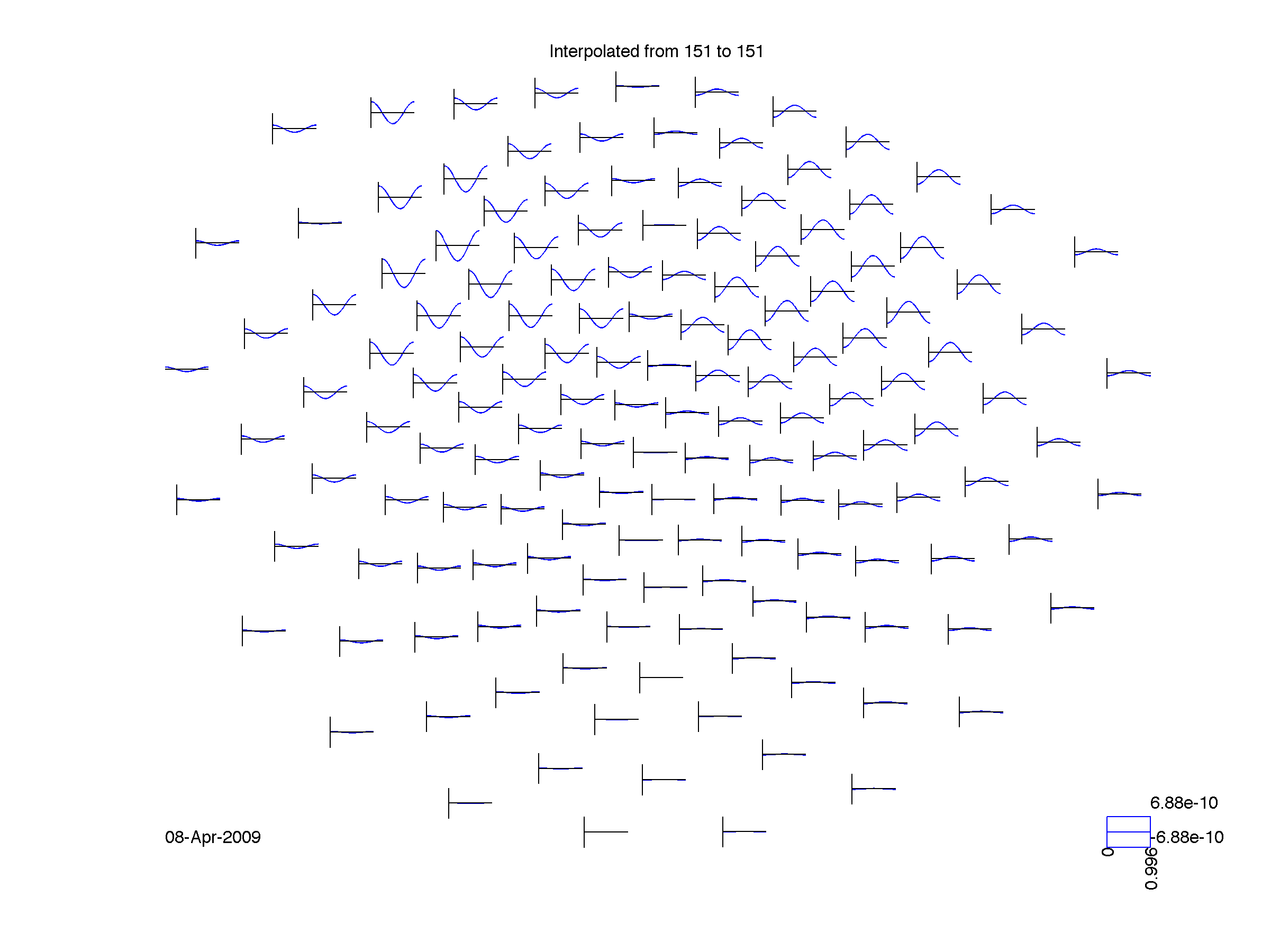``````figure; ft_multiplotER(cfg, avg275_275);
title('Interpolated from 151 to 151');
orient portrait; print -dpng -r300 avg275_275.png
``````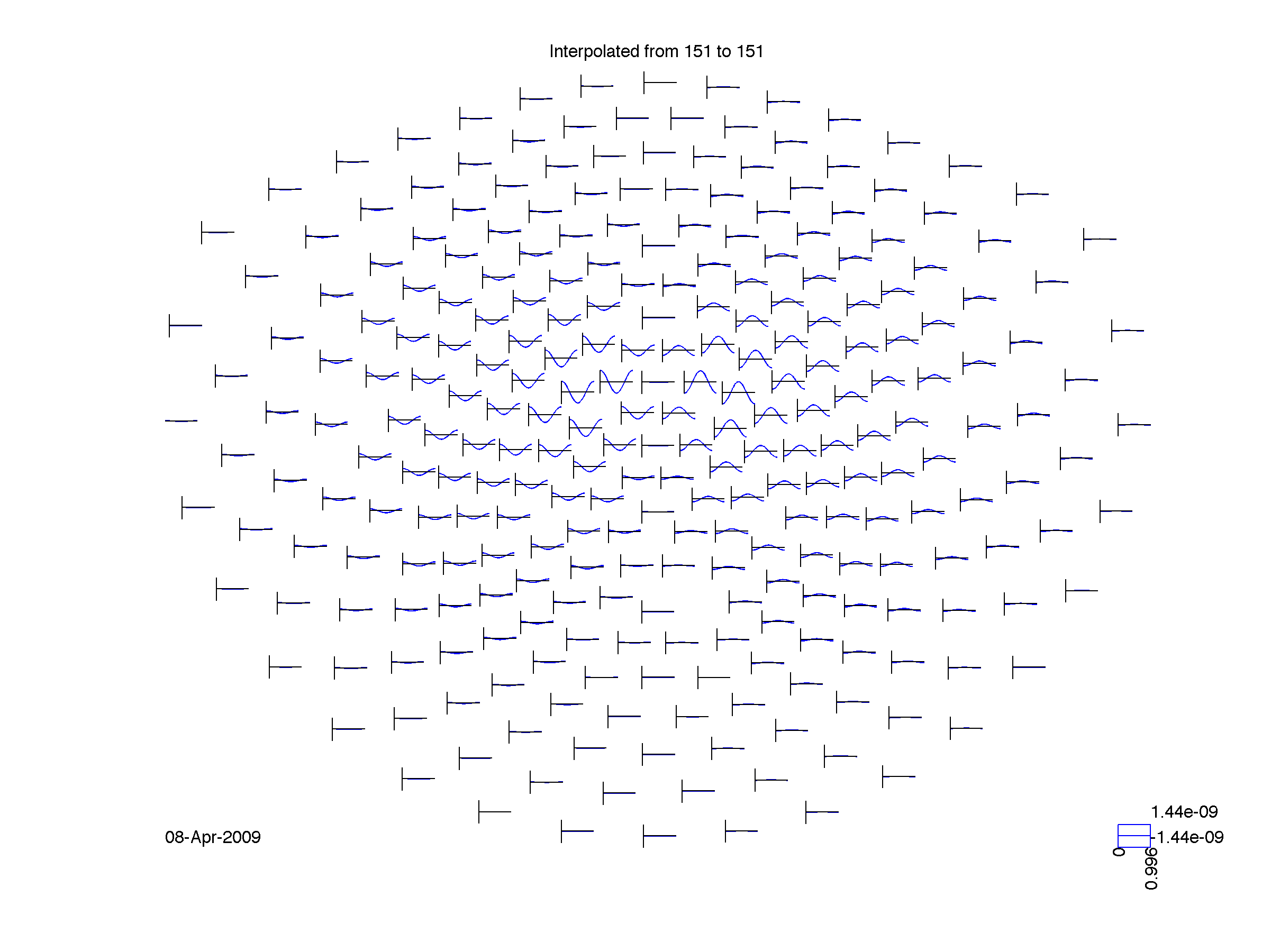``````% now plot them together
cfg = [];
figure; ft_multiplotER(cfg, avg151, avg151_151, avg275_151);
orient portrait; print -dpng -r300 compare151.png
``````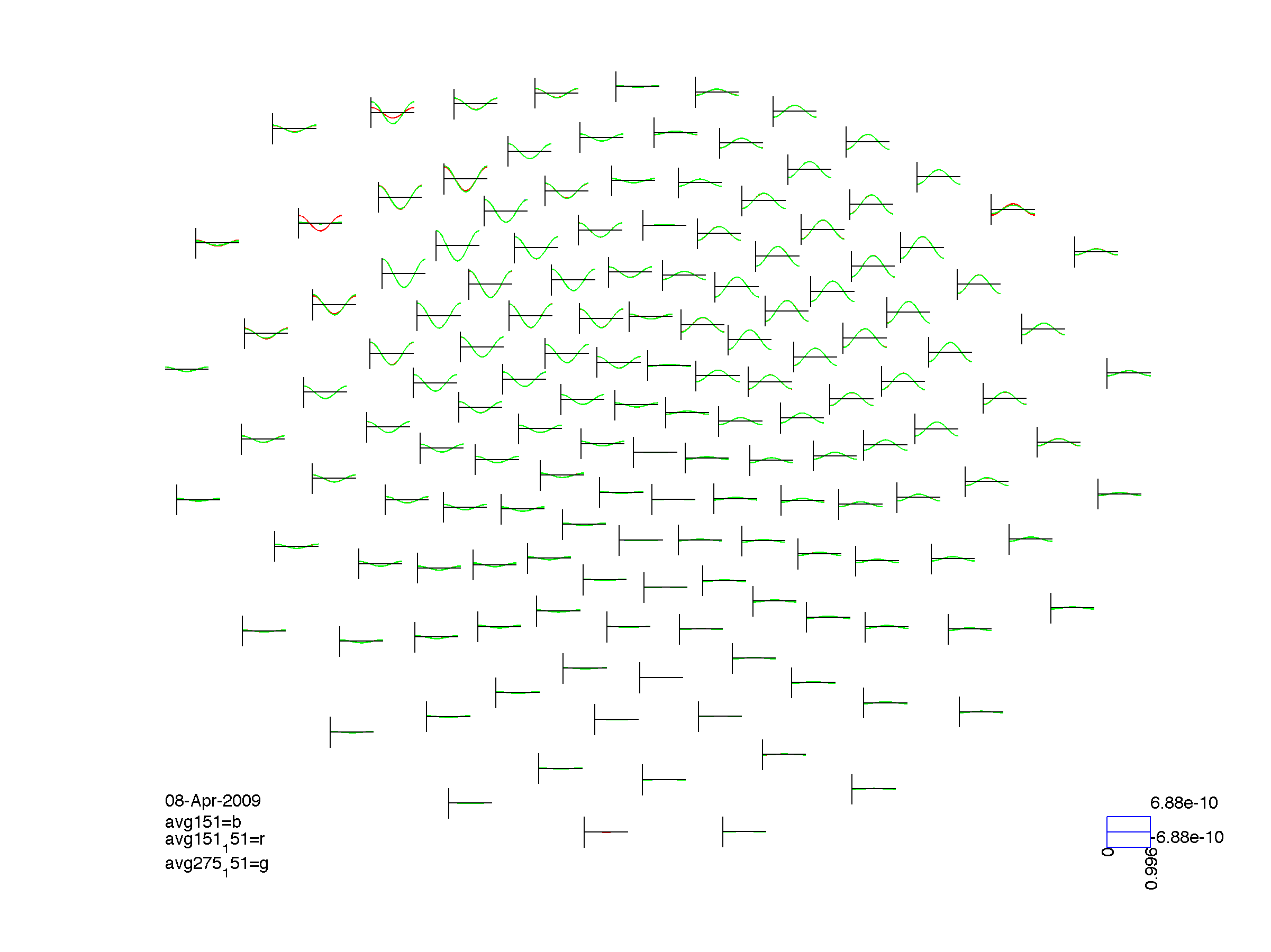``````figure; ft_multiplotER(cfg, avg275, avg275_275, avg151_275);
orient portrait; print -dpng -r300 compare275.png
``````